EngliSea > M > math > ￤references > Luffa

Glome, Hollow Glome and Luffa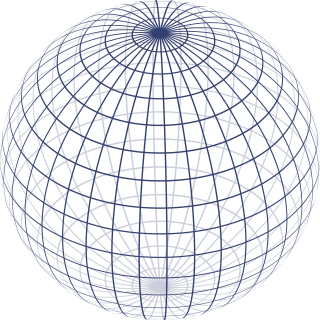3-sphere
Any loop or circular path on the 3-sphere can be continuously shrunk to a point without leaving the 3-sphere. The Poincaré conjecture provides that the 3-sphere is the only three-dimensional manifold (up to homeomorphism) with these properties.
3447

Cartesian product
For sets A and B, the Cartesian product A × B is the set of all ordered pairs(a, b) where a ∈ A and b ∈ B.
3619

Closed set
A set which contains all its limit points. A closed set contains its own boundary.
3476

Compact space
Compactness is a property that generalizes the notion of a subset of Euclidean space being closed (that is, containing all its limit points) and bounded(that is, having all its points lie within some fixed distance of each other).
3494

Connected space
A topological space X is said to be disconnected if it is the union of two disjoint nonempty open sets. Otherwise, X is said to be connected.
3492

Contractible space
In mathematics, a topological space X is contractible if the identity map on X is null-homotopic, i.e. if it is homotopic to some constant map.
3578

Glome in colors 01
What is the shape of the universe? Does it extend infinitely? We can go around the earth forever but can not find the end. Likewise, the universe may be finite but endless. Let's consider a mathematical model suitable for such a space. A summary of Eucli ...
707 COMMENT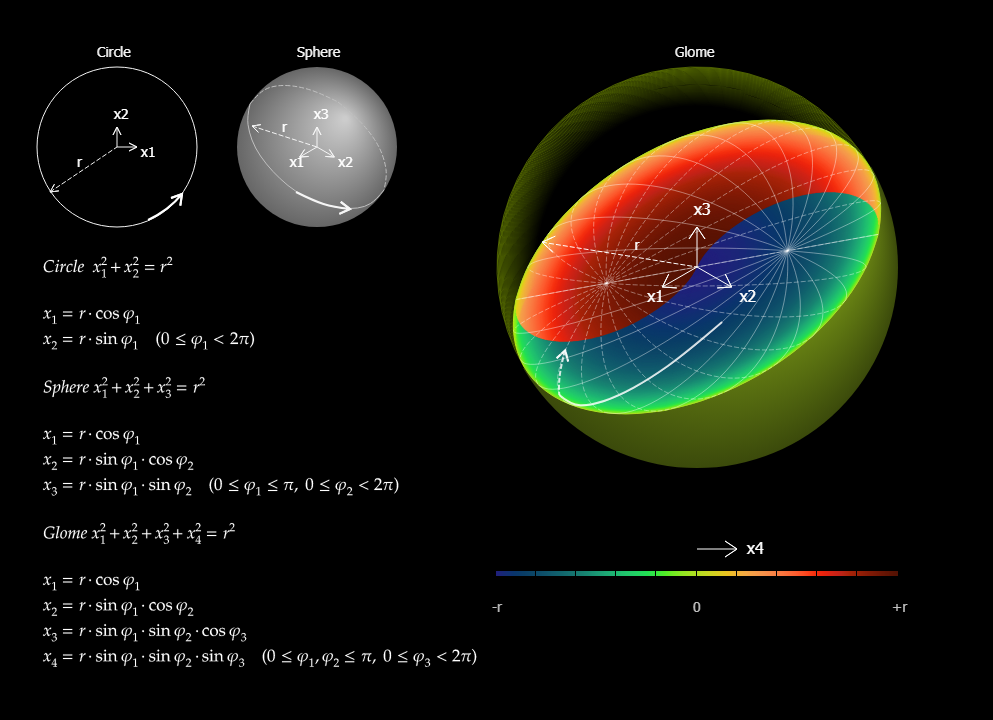Glome in colors 02
3677 COMMENT

Glome in colors 03
In a one-dimensional space, there is only one direction. So it is straight. If a one-dimensional space is looped, the relationship between points can be represented by a closed curve. But the curve shows just the relationship between points, not the shape ...
9044 COMMENT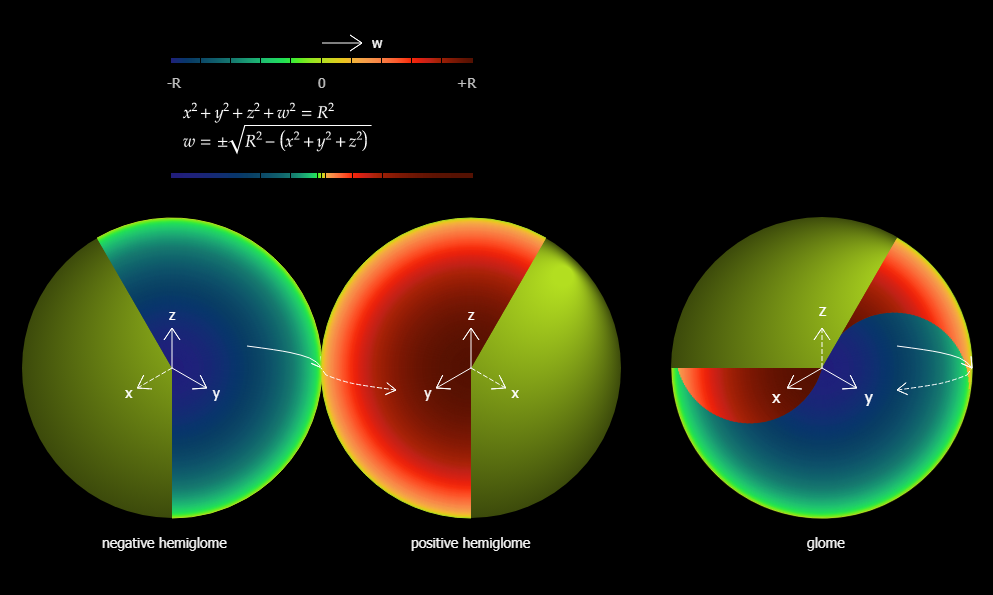Glome in colors 04
All paths are reflected at the boundary of the glome making the space bounded without a boundary. Every path passing through any point in the glome is continuous at the point even at the boundary. This is analogous to the projection of a sphere.
9045 COMMENT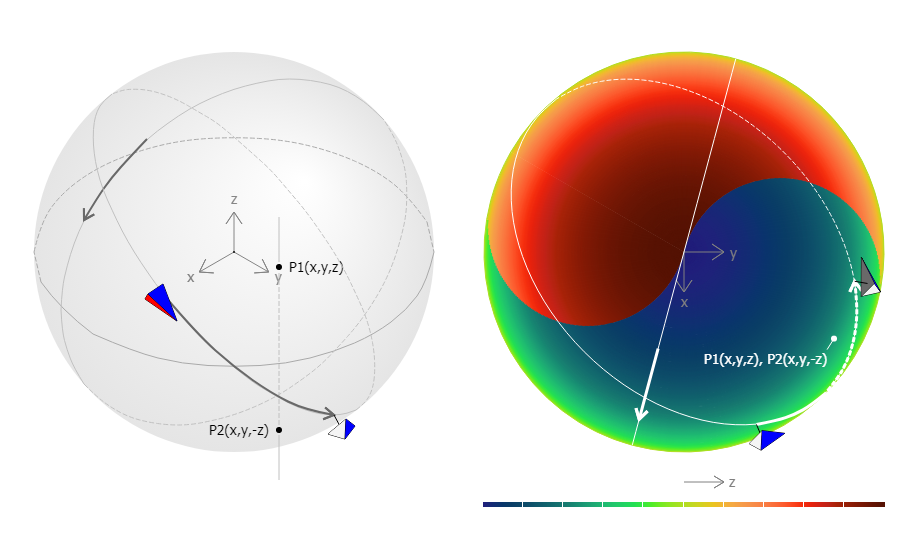Glome in colors 05
9047 COMMENT

Glome in colors 06
In the projection of a sphere, each point on the disc except the circumference represents two points. One is a point on the upper hemisphere and the other is a point on the lower hemisphere. Likewise, each point in the glome except the surface represents ...
9048 COMMENT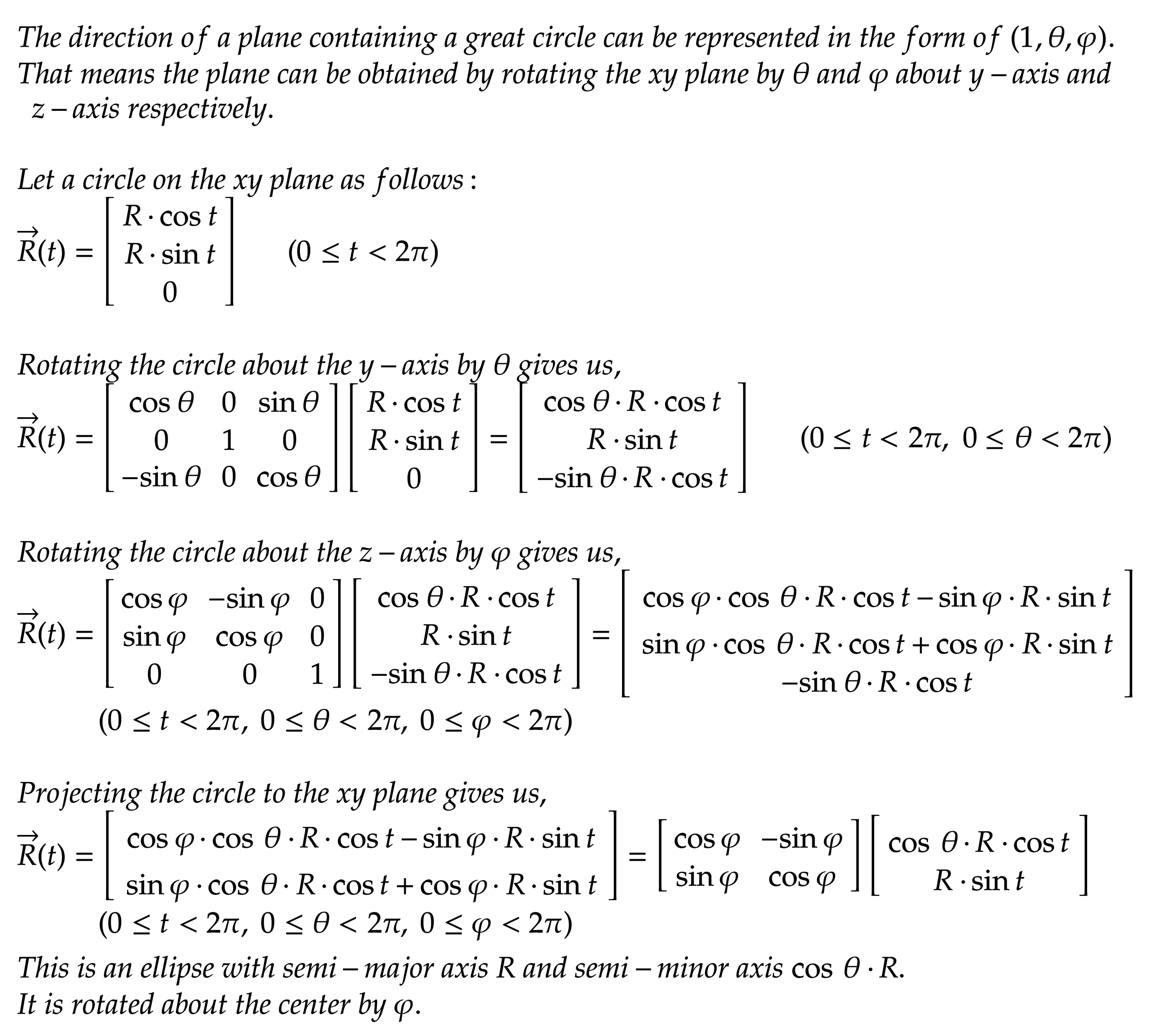Glome in colors 07
9049 COMMENT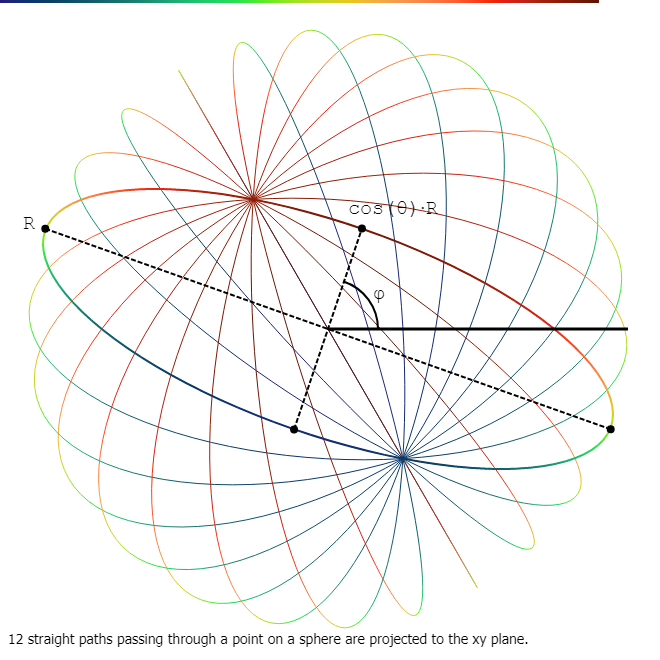Glome in colors 08
9050 COMMENT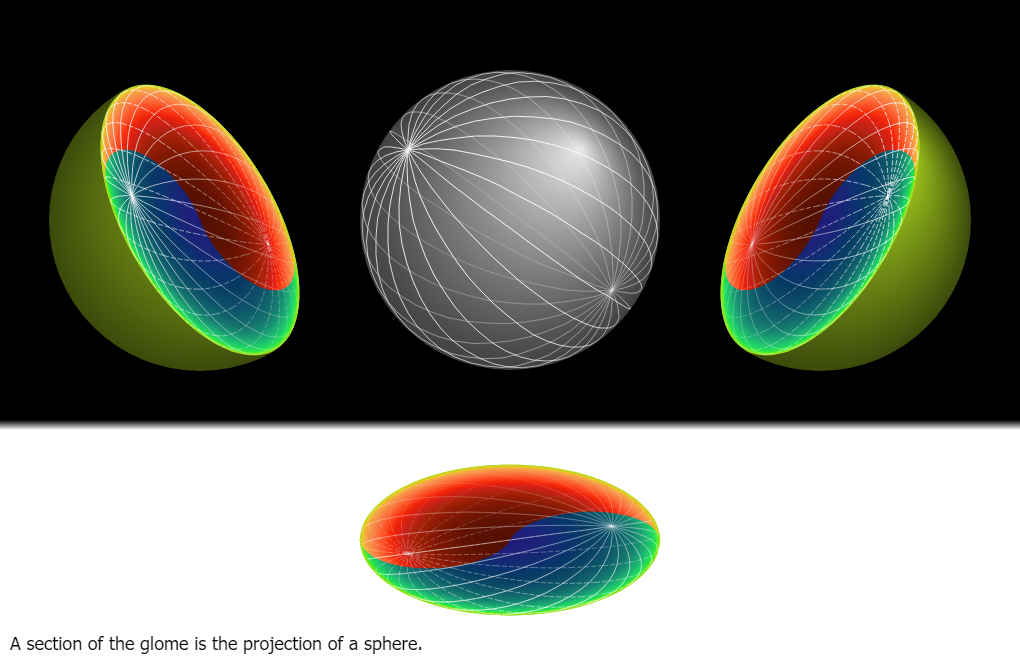Glome in colors 09
In a glome, the section where z is zero is the projection of a sphere.
9051 COMMENT

Glome in colors 10
Going straight is represented by following an ellipse on the great section and the movement from one hemiglome to another is represented by the contact between the ellipse and the circumference of the great section. For every direction at any point in a g ...
9052 COMMENT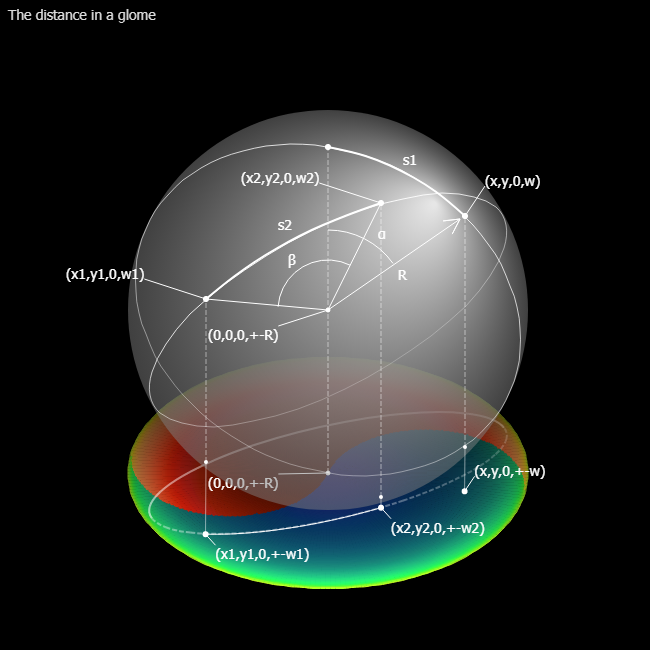Glome in colors 11
9053 COMMENT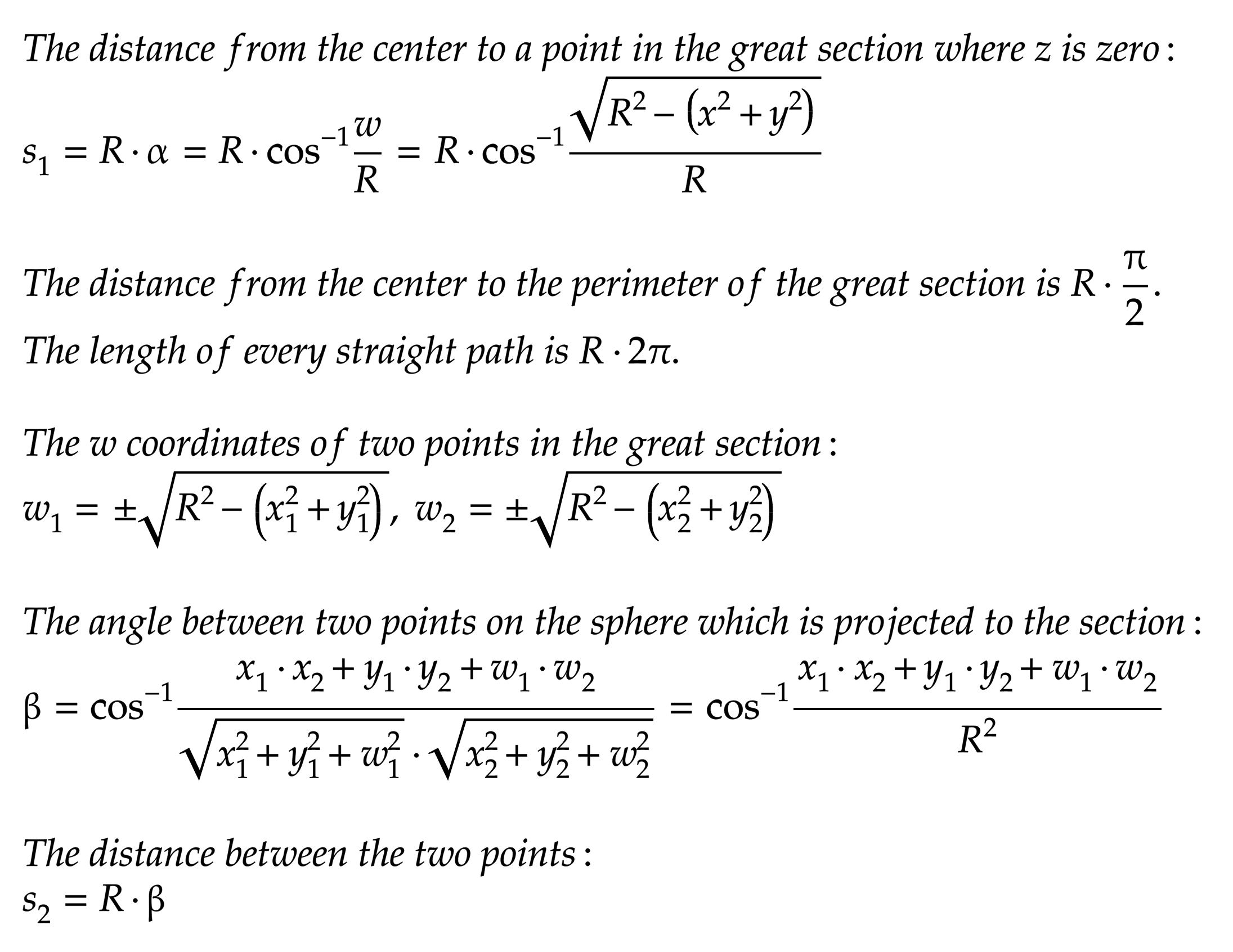Glome in colors 12
9055 COMMENT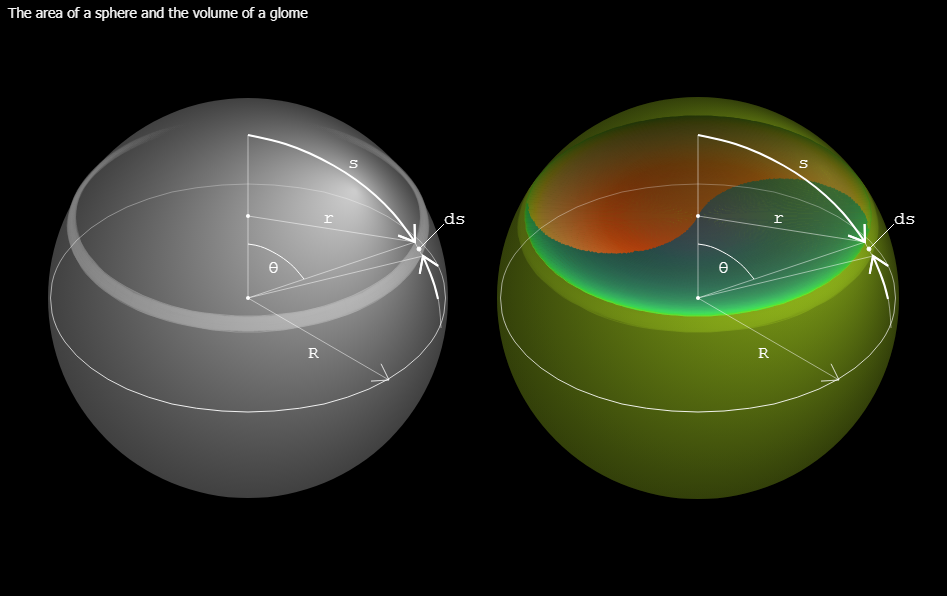Glome in colors 13
9056 COMMENT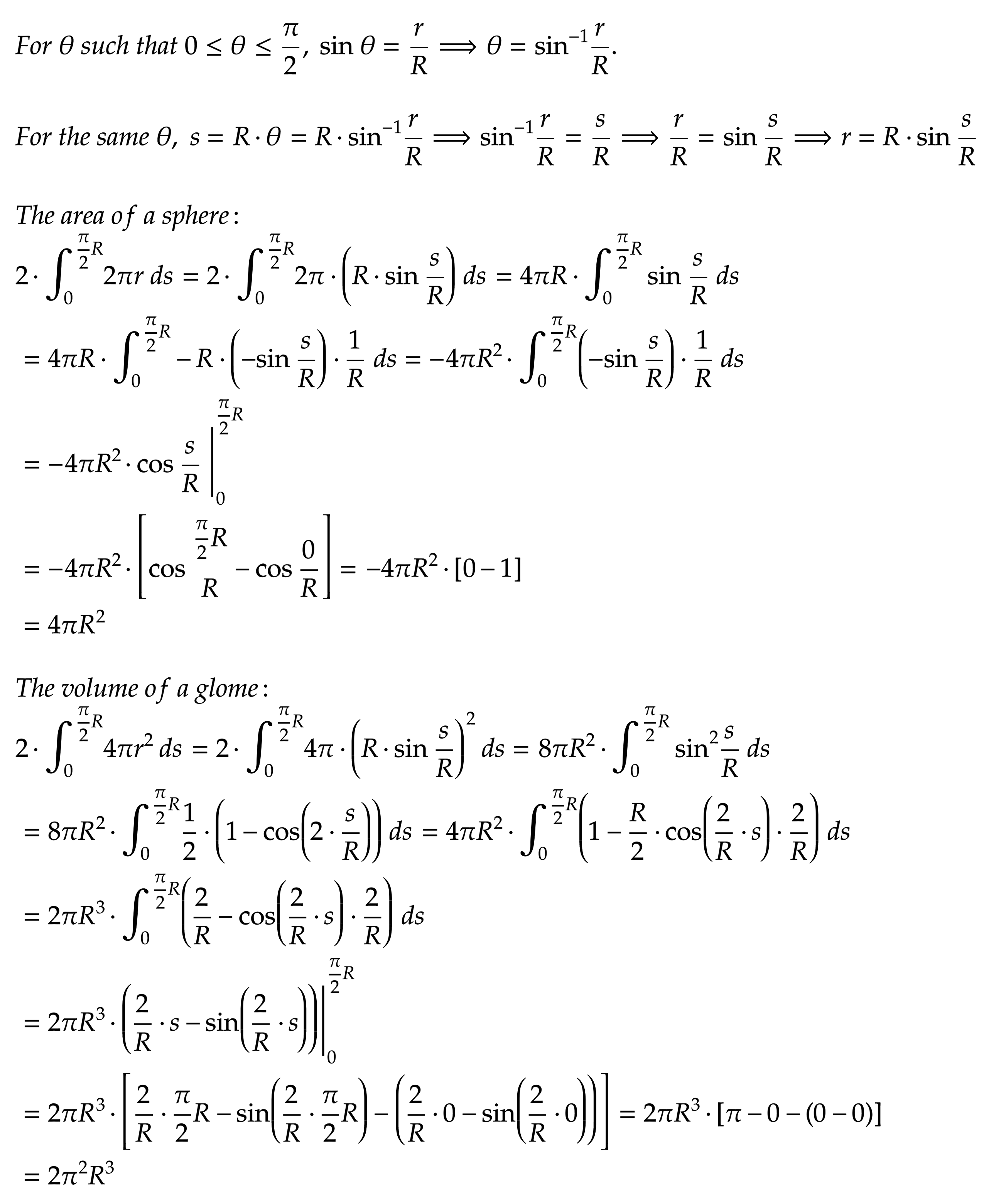Glome in colors 14
9057 COMMENT

Glome in colors 15
A circular model has two dimensions, but the space represented by the model has only one dimension. The spheric model has three dimensions, but there are only two dimensions in the space that the model represents. Likewise, the space represented by the gl ...
9058 COMMENT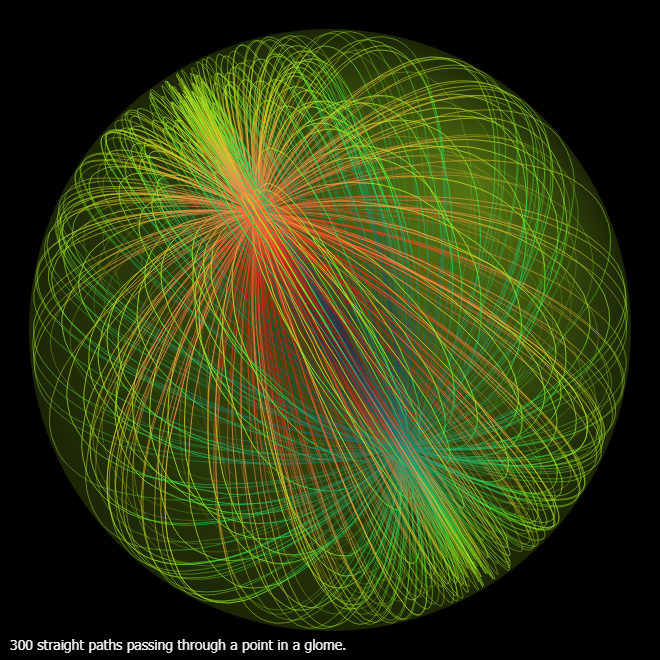Glome in colors 16
9059 COMMENT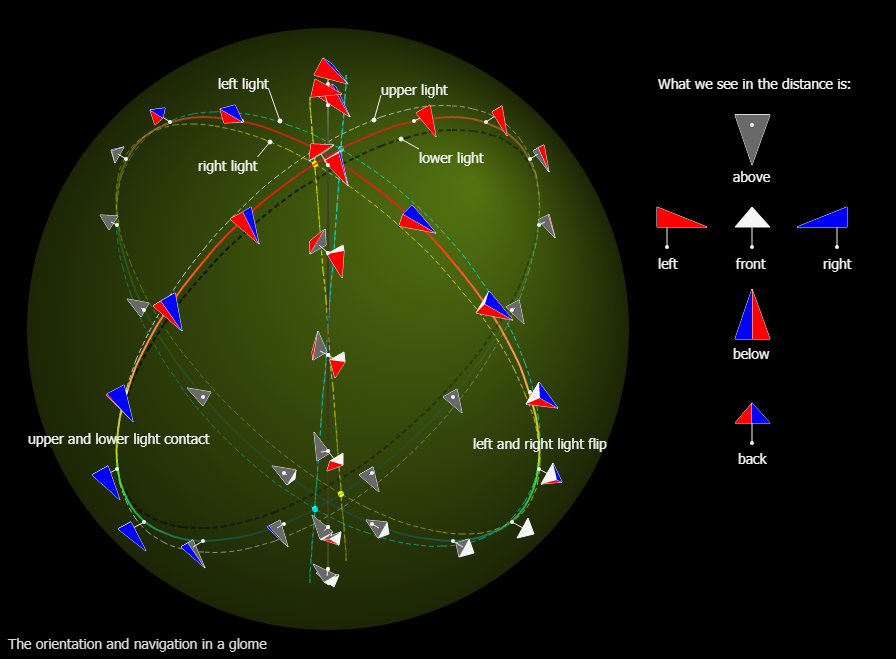Glome in colors 17
9060 COMMENT

Glome in colors 18
For any two points in a glome, there is a plane containing the two points and the origin. By cutting the glome with the plane we get a great section where we can draw a great ellipse passing through the two points. This means that at any point in a glome ...
9061 COMMENT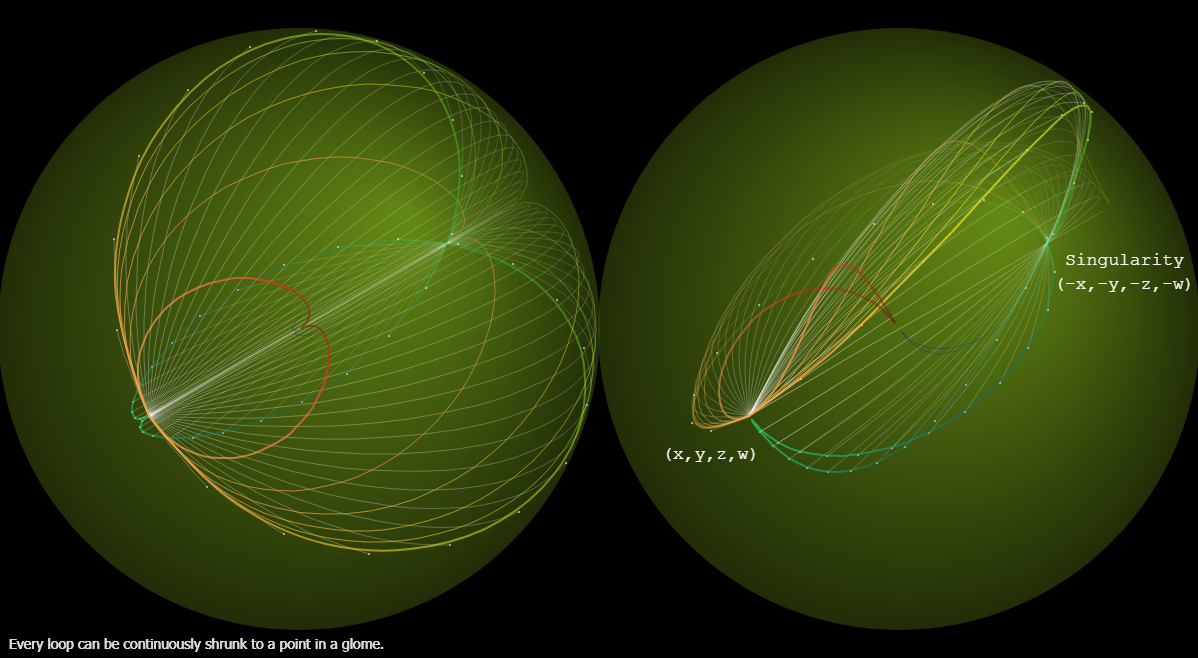Glome in colors 19
9062 COMMENT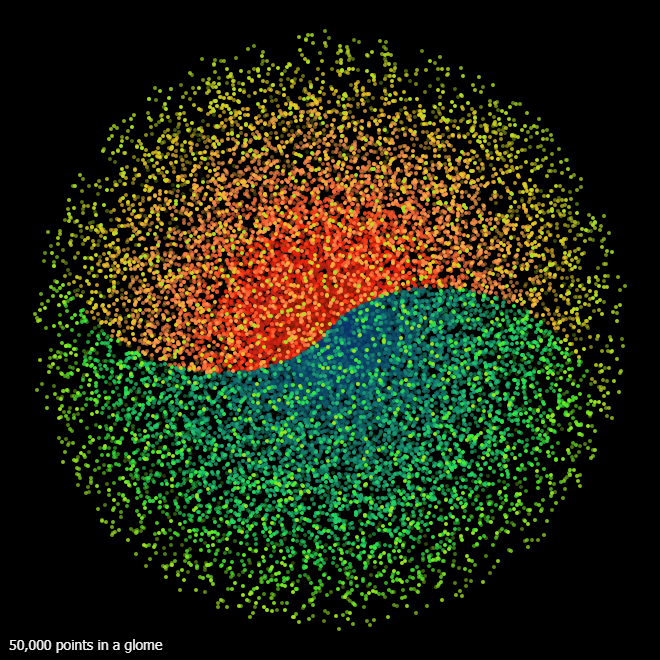Glome in colors 20
9063 COMMENT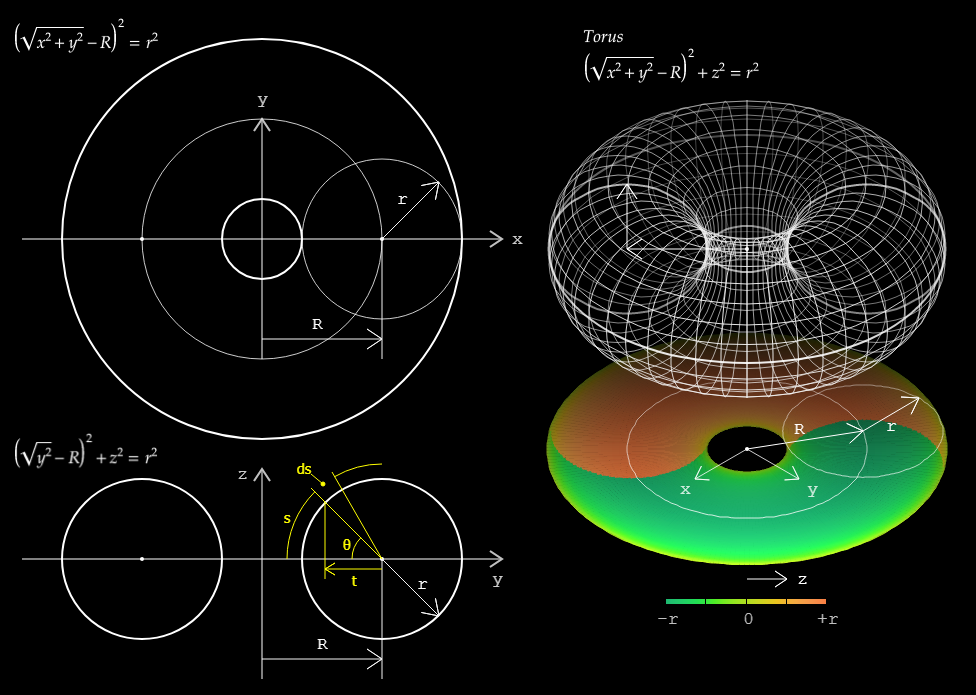Hollow Glome and Luffa 01
9064 COMMENT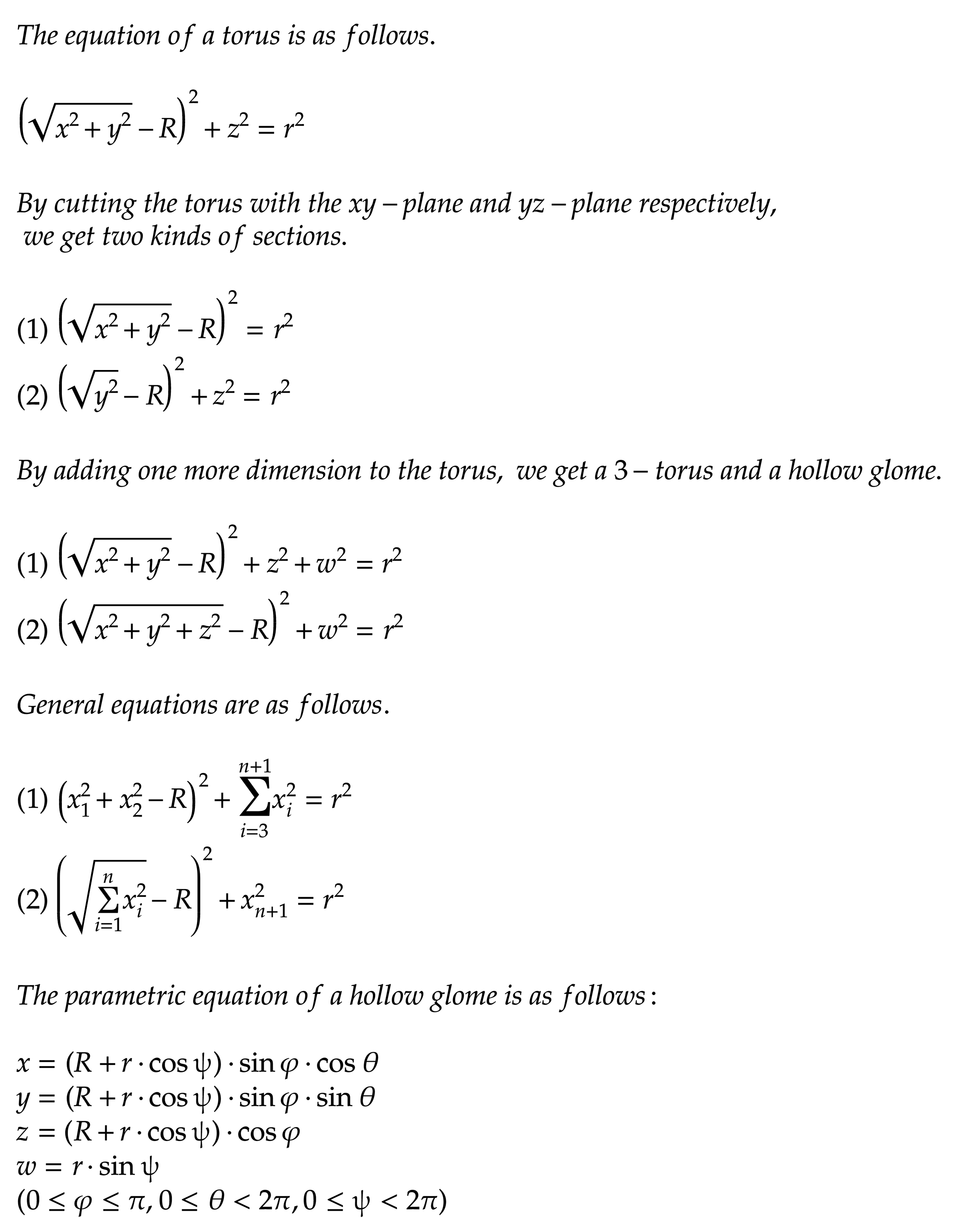Hollow Glome and Luffa 02
9065 COMMENT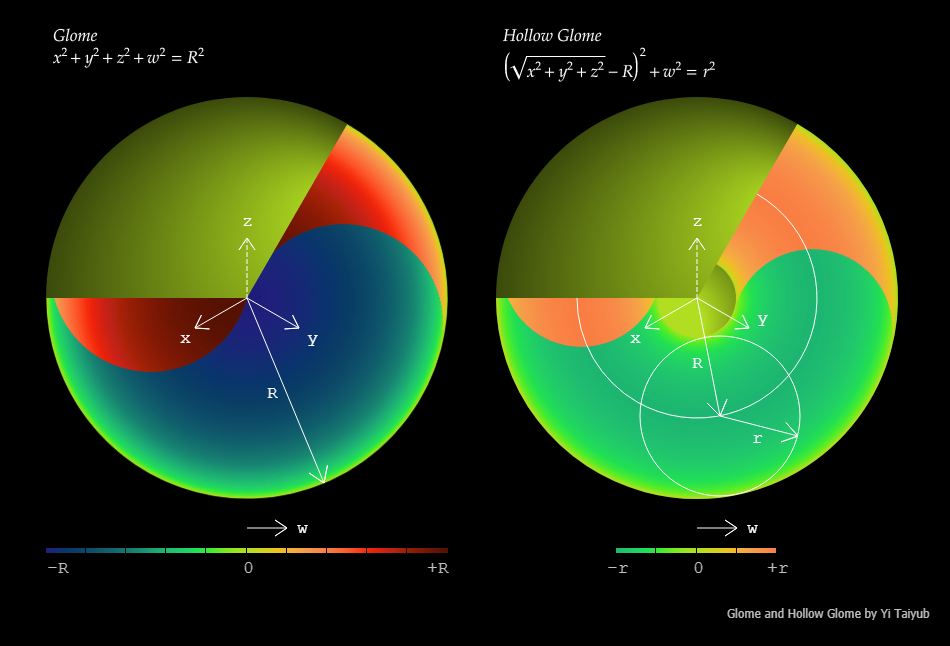Hollow Glome and Luffa 03
9066 COMMENT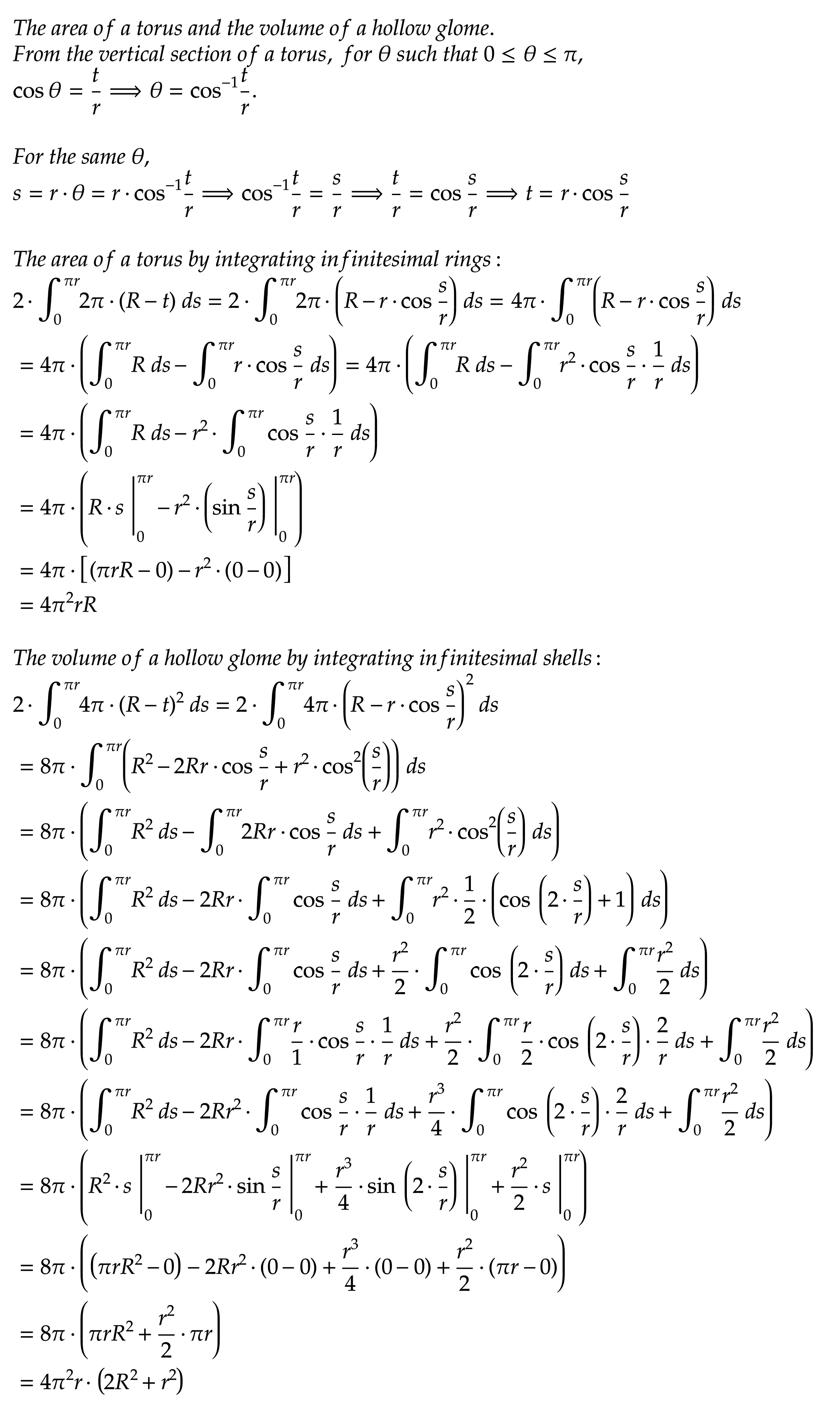Hollow Glome and Luffa 04
9067 COMMENT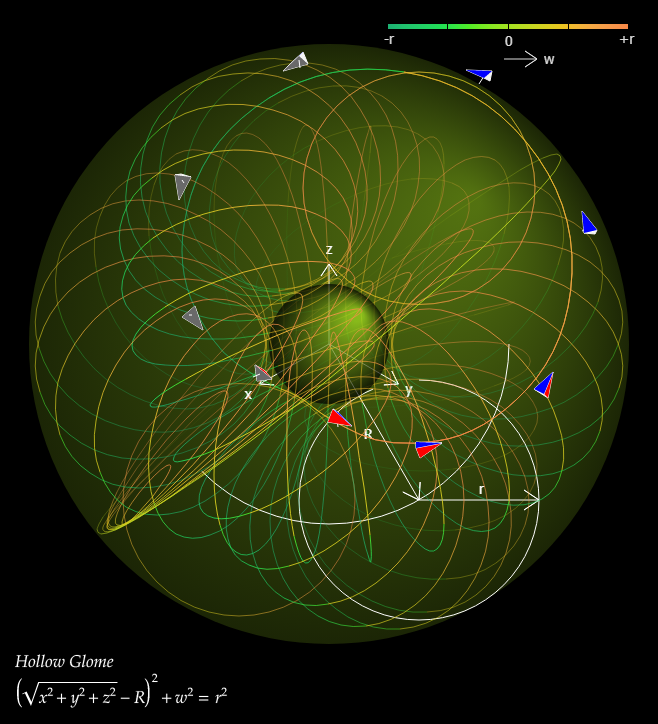Hollow Glome and Luffa 05
9068 COMMENT

Hollow Glome and Luffa 06
Suppose a three-dimensional space which is bounded and path-connected. Let the space contain holes. Let f and g be continuous functions taking this space as a domain. f(x,y,z) is equal to g(x,y,z) at the boundary of the space and is different at the other ...
9069 COMMENT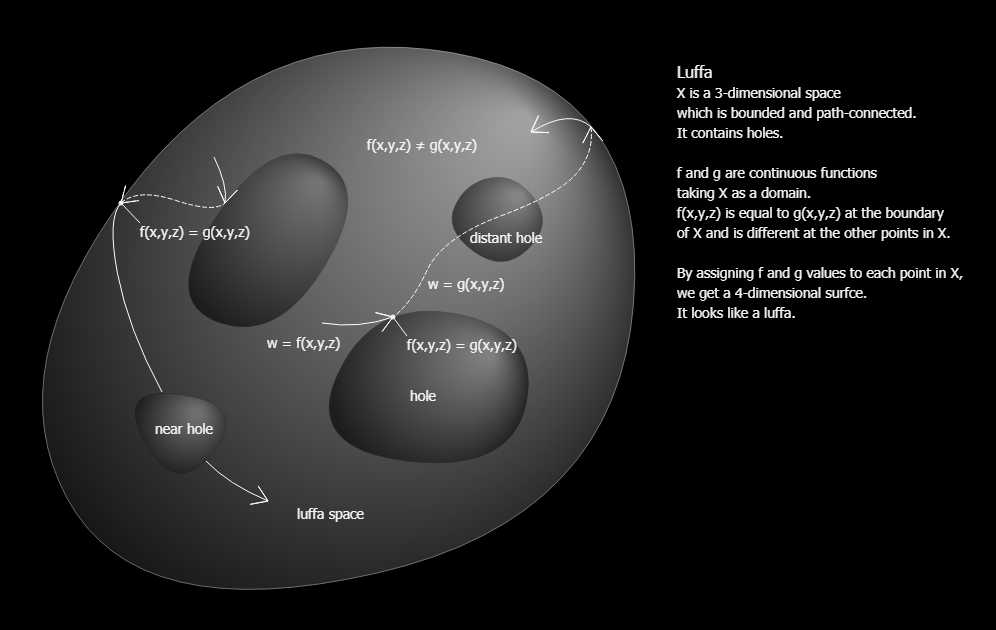Hollow Glome and Luffa 07
9070 COMMENT

Implicit Function
2018-12-02 08:49
3572

Implicit function theorem
2018-12-02 09:00
3651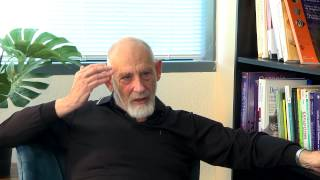Leonard Susskind
The space itself may be more than three dimensions. But we can't visualize more dimensions. The architecture of the brain itself is evolved in the world of three dimensions. We only describe more dimensions by pure mathematics.
5242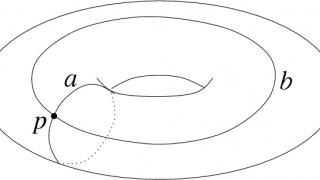◌◌◌ Loop
A loop in mathematics, in a topological space X is a continuous function f from the unit interval I = [0,1] to X such that f(0) = f(1).
3450n-ball
A set of points whose distance from a particular point is less than a specified length in n-dimensional space.
3445n-sphere
A set of points at a specified distance from a particular point in (n+1)-dimensional space. The pair of points at the ends of a line segment is a 0-sphere.
3446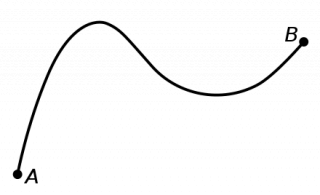◌◌◌ Path
In mathematics, a path in a topological space X is a continuous function f from the unit interval I = [0,1] to X f : I → X. A topological space for which there exists a path connecting any two points is said to be path-connected.
3453

Poincaré conjecture
Every simply connected, closed 3-manifold is homeomorphic to the 3-sphere.
3579

Real coordinate space
In mathematics, real coordinate space of n dimensions, written Rn (/ɑːrˈɛn/ ar-EN) (also written ℝn with blackboard bold) is a coordinate space that allows several (n) real variables to be treated as a single variable.
3495

Simply connected space
A topological space X is called simply connected if it is path-connected and any loop in X defined by f : S1 → X can be contracted to a point.
3534

Simply connected space
A sphere (or, equivalently, a rubber ball with a hollow center) is simply connected, because any loop on the surface of a sphere can contract to a point, even though it has a "hole" in the hollow center.
3577Singularities Explained | Infinite Series
Singularities Explained | Infinite Series
3563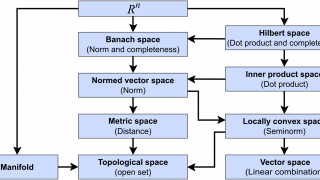◌◌◌ Space
A space consists of selected mathematical objects that are treated as points, and selected relationships between these points.
5251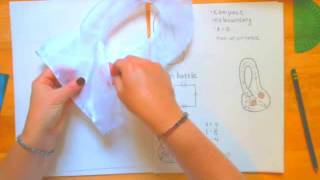The Poincare Conjecture
In the early 1900s mathematicians and physicists were very interested in the shape of space. New experiments and theories were being developed that would ultimately create relativity theory and change our entire view of the universe.
3533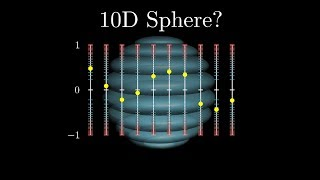Thinking visually about higher dimensions
Thinking visually about higher dimensions
3388

Three-torus
The three-dimensional torus, or triple torus, is defined as the Cartesian product of three circles. The triple torus is a three-dimensional compact manifold with no boundary.
3612

Topological manifold
A topological space which locally resembles real n-dimensional space in a sense defined below.
3493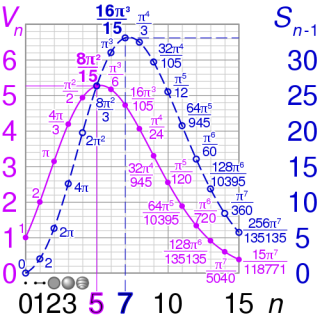volumes and surface areas of n-spheres
Graphs of volumes and surface areas of n-spheres of radius 1.
3448-